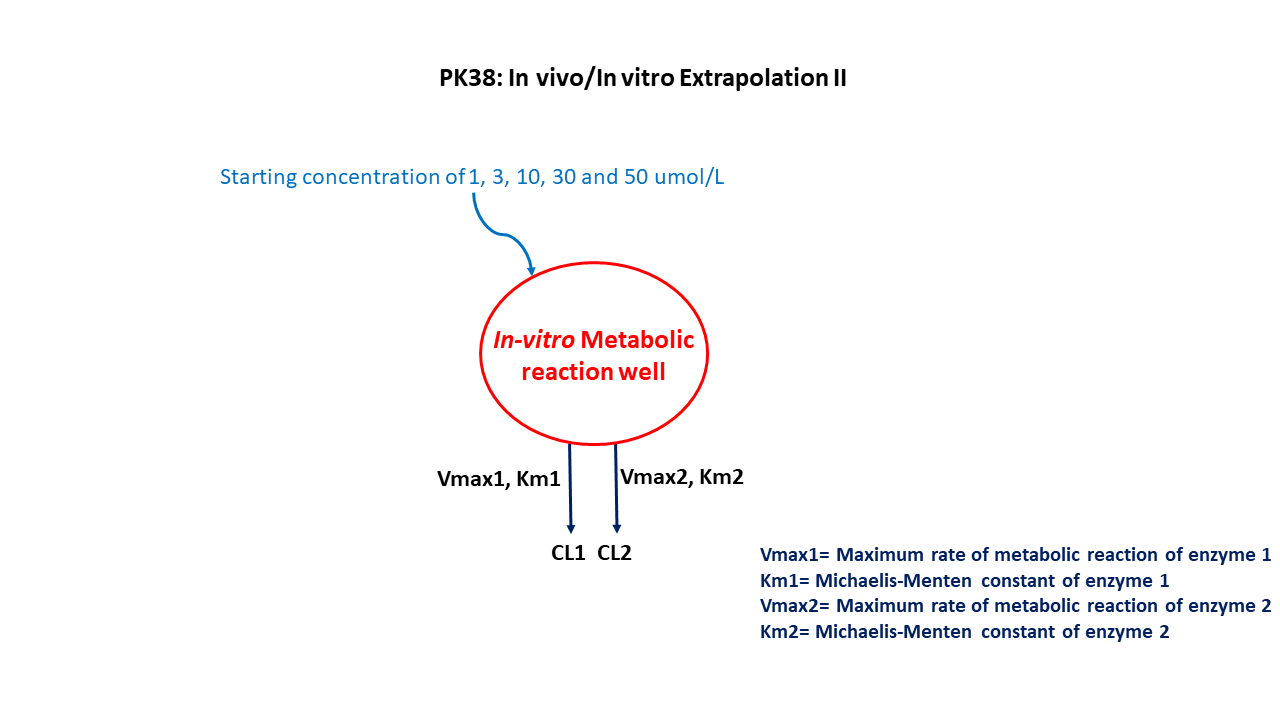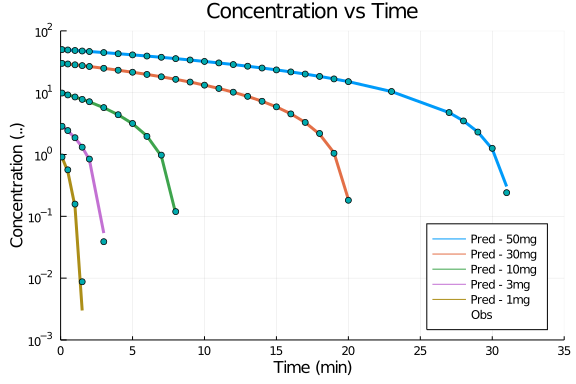# Exercise PK38 - Invitro/invivo extrapolation II

### Background

• Structural Model - Two Enzyme Model

• Route of admnistration - in vitro experiment

• Dosage regimen - 50,30,10,3,1 μmol### Learning Outcome

To analysze the data based on the metabolic rate of compound using diffenrential equation instead of rather than transforming data into rate versus concentration plot.

### Objectives

• To fit a two enzyme model to a concentration-time data

### Libraries

call the "necessary" libraries to get started.

using Pumas
using Plots
using CSV
using StatsPlots
using Random


### Model

pk_38        = @model begin
@param begin
tvvmax1  ∈ RealDomain(lower=0)
tvkm1    ∈ RealDomain(lower=0)
tvvmax2  ∈ RealDomain(lower=0)
tvkm2    ∈ RealDomain(lower=0)
Ω        ∈ PDiagDomain(4)
end

@random begin
η        ~ MvNormal(Ω)
end

@pre begin
Vmax1    = tvvmax1 * exp(η)
Km1      = tvkm1 * exp(η)
Vmax2    = tvvmax2 * exp(η)
Km2      = tvkm2 * exp(η)
Vmedium  = 1
end

@vars begin
VMKM    := ((Vmax1*Central/(Km1+Central))+(Vmax2*Central/(Km2+Central)))
end

@dynamics begin
Central' =  -VMKM/Vmedium
end

@derived begin
cp       = @. Central/Vmedium
end
end

PumasModel
Parameters: tvvmax1, tvkm1, tvvmax2, tvkm2, Ω, σ_add
Random effects: η
Covariates:
Dynamical variables: Central
Derived: cp, dv
Observed: cp, dv


### Parameters

Parameters provided for simulation are as below. tv represents the typical value for parameters.

• Vmax1 - Maximum Metabolic Capacity of System 1 (nmol/min)

• Km1 - Michelis Menten Constant of System 1 (μmol/l)

• Vmax2 - Maximum Metabolic Capacity of System 2 (nmol/min)

• Km2 - Michelis Menten Constant of System 2 (μmol/l)

param = ( tvvmax1 = 0.960225,
tvkm1   = 0.0896,
tvvmax2 = 1.01877,
tvkm2   = 8.67998,
Ω       = Diagonal([0.0,0.0,0.0,0.0]),

(tvvmax1 = 0.960225, tvkm1 = 0.0896, tvvmax2 = 1.01877, tvkm2 = 8.67998, Ω
= [0.0 0.0 0.0 0.0; 0.0 0.0 0.0 0.0; 0.0 0.0 0.0 0.0; 0.0 0.0 0.0 0.0], σ_a
dd = 0.02)


### Dosage Regimen

Each group is given a different conc of 50, 30, 10,3 & 5 μmol/L

ev1      = DosageRegimen(50, time=0, cmt=1)
sub1     = Subject(id=1, events=ev1, time=[0.1,0.5,1,1.5,2,3,4,5,6,7,8,9,10,11,12,13,14,15,16,17,18,19,20,23,27,28,29,30,31])
ev2      = DosageRegimen(30, time=0, cmt=1)
sub2     = Subject(id=2, events=ev2, time=[0.1,0.5,1,1.5,2,3,4,5,6,7,8,9,10,11,12,13,14,15,16,17,18,19,20])
ev3      = DosageRegimen(10, time=0, cmt=1)
sub3     = Subject(id=3, events=ev3, time=[0.1,0.5,1,1.5,2,3,4,5,6,7,8])
ev4      = DosageRegimen(3, time=0, cmt=1)
sub4     = Subject(id=4, events=ev4, time=[0.1,0.5,1,1.5,2,3])
ev5      = DosageRegimen(1, time=0, cmt=1)
sub5     = Subject(id=5, events=ev5, time=[0.1,0.5,1,1.5])
pop5_sub = [sub1,sub2,sub3,sub4,sub5]

Population
Subjects: 5
Covariates:


### Simulation

We will simulate the concentration for the pre-specified time point for each group

Random.seed!(123)
sim_pop5_sub = simobs(pk_38, pop5_sub, param)
df1          = DataFrame(sim_pop5_sub)


### Dataframe and Plots

Use the dataframe for plotting

df_id1_dv = filter(x -> x.id == "1", df1)
df_id2_dv = filter(x -> x.id == "2", df1)
df_id3_dv = filter(x -> x.id == "3", df1)
df_id4_dv = filter(x -> x.id == "4", df1)
df_id5_dv = filter(x -> x.id == "5", df1)

@df df_id1_dv plot(:time, :cp, yaxis=:log, linewidth=3, legend=:bottomright,
title="Concentration vs Time", label="Pred - 50mg",
xlabel="Time (min)", ylabel="Concentration (..)",
xticks=[0,5,10,15,20,25,30,35], xlims=(0,35),
yticks=[0.001,0.01,0.1,1,10,100], ylims=(0.001,100))
@df df_id2_dv plot!(:time, :cp, label="Pred - 30mg", linewidth=3)
@df df_id3_dv plot!(:time, :cp, label="Pred - 10mg", linewidth=3)
@df df_id4_dv plot!(:time, :cp, label="Pred - 3mg", linewidth=3)
@df df_id5_dv plot!(:time, :cp, label="Pred - 1mg", linewidth=3)
@df df1 scatter!(:time, :dv, label="Obs")